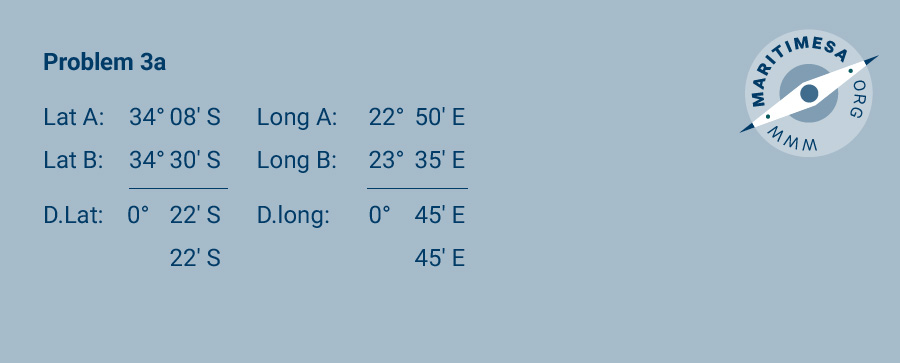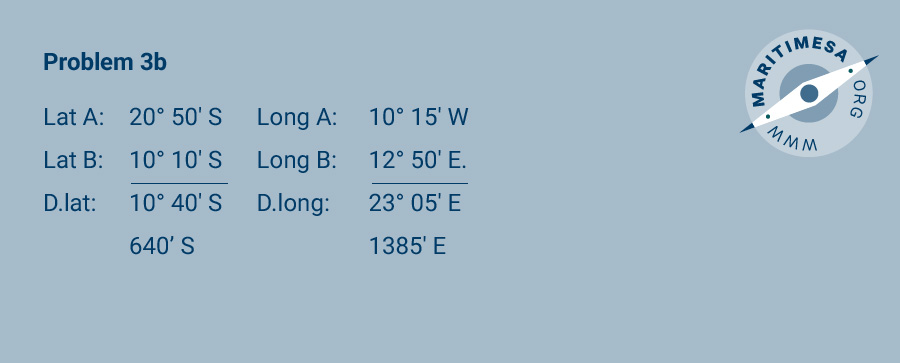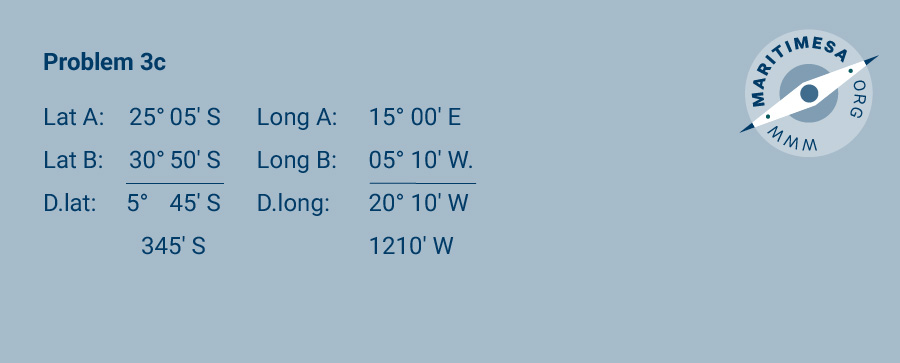# Measurement of position and distance on the earth’s surface

So far we have covered the basic definitions and concepts used in nautical navigation. We will now look at a number of simple problems to demonstrate our understanding of these definitions/concepts. This practical assignment assumes that you have access to a physical copy of a nautical chart for the Table Bay and False Bay areas.

Measurement of position.

Problem 1. What is the latitude and longitude of the following points:

1. Cape Point Lighthouse.
2. Slangkoppunt Lighthouse.
3. Cape Hangklip Lighthouse.

Problem 2. Plot the following positions on your chart:

1. 34° 10’ S 18° 15’ E.
2. 33° 50’ S 18° 25’ E.
3. 34° 25’ S 18° 35’ E.

Problem 3. What is the difference in latitude (d.lat) and the difference in longitude (d.long) between the following positions:

1. Pos A : 34° 08’ S 22° 50’ E
Pos B : 34° 30’ S 23° 35’ E.
2. Pos A : 20° 50’ S 10° 15’ W
Pos B : 10° 10’ S 12° 50’ E.
3. Pos A : 25° 05’ S 15° 00’ E
Pos B : 30° 50’ S05° 10’ W.

Measuring distance.

Problem 4. What is the distance in nautical miles between the following positions:

1. Roman Rock Light and Cape Hangklip Light.
2. Cape Point Light and position 34° 24’ S 18° 15’ E.
3. Position 34° 00’ S 18° 10’ E and position 34° 20’ S 18° 18’ E.

Transferring a position from one chart to another. When transferring a position from one chart to another it should preferably be done using a bearing and range of a conspicuous point common to both charts, ie lighthouses, point of land, man made structure, geographical feature. The use of latitude and longitude should be avoided because the graduations on the two may differ.

Relationship between speed , time and distance. The relationship between time, distance and speed can best be illustrated by the following formula:

Speed (in knots) = Distance (in nautical miles)/Time (in hours)

To demonstrate the above the following simple problems are given:

Problem 5. What is the distance (in nautical miles) covered by a ship steaming at 12 knots for 23 hours and 45 minutes?

Problem 6. What is the speed of a ship (in knots) if it has covered 350 nautical miles in 19 hours and 15 minutes?

Problem 7. How long (in hours and minutes) will a ship take to steam between position A (34° 18’ S 18° 40’ E) and position B (34° 20’ S 18° 10’ E) at 8 knots?

Problem 1

1. 34° 21.4’ S 18° 29.8’ E.
2. 34° 08.9’ S 18° 19.2’ E.
3. 34° 23.2’ S 18° 49.7’ E.

Problem 2

Problem 3Problem 4

1. 22.0 miles.
2. 12.7 miles.
3. 21.0 miles.

Problem 5
Distance = 23.75 x 12 = 285 miles.

Problem 6
Speed = 350/19.25 = 18.2 knots.

Problem 7
Time = 26.9/8 = 3 hours 22 minutes